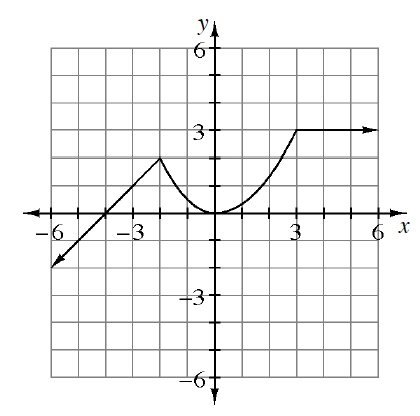### Home > PC3 > Chapter 11 > Lesson 11.1.2 > Problem11-33

11-33.Using the graph of $y = f (x)$ at right, sketch each of the following transformations.

1. $y = f(x) + 2$

The function value, $f(x)$, is the $y$-value. How does the graph change if $2$ is added to each $y$-value?

2. $y = 2f(x + 1)$

New function: If $x = 1$, f(new) is equivalent to $f(2)$ from the original function. Also, what does multiplication by $2$ do to the function?

3. $y = −f(x − 2)$

$f(x)$ became $−f(x)$, or y became $−y$. Also, be sure to shift the curve $2$ units right.

4. $y = f(2x)$

$f(8)$ in the original function occurs when $x = 4$ in the new function.